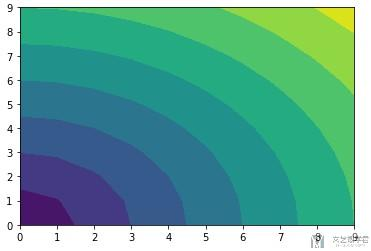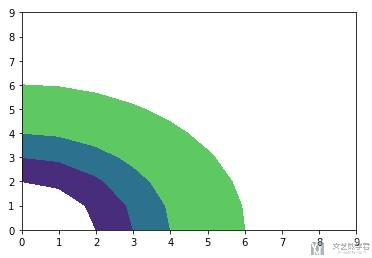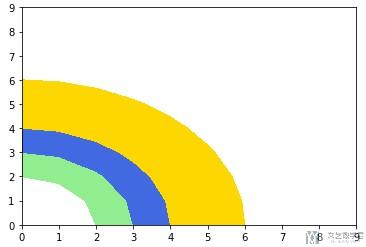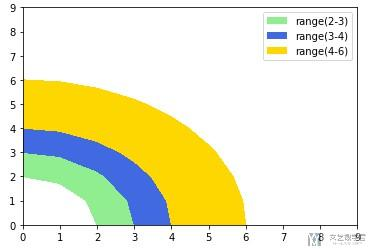# matplotlib绘图优化-绘制轮廓图和进行填充(contourf)

• A+

## 简介

contourf函数的官方文档: matplotlib.pyplot.contourf

## 绘图

### levels的作用

1. import numpy as np
2. import matplotlib.pyplot as plt
3. x, y = np.meshgrid(np.arange(10),np.arange(10))
4. z = np.sqrt(x**2 + y**2)
5. cs = plt.contourf(x,y,z,levels=10)
6. plt.show()1. import numpy as np
2. import matplotlib.pyplot as plt
3. x, y = np.meshgrid(np.arange(10),np.arange(10))
4. z = np.sqrt(x**2 + y**2)
5. cs = plt.contourf(x,y,z,levels=[2,3,4,6])
6. plt.show()### 自定义颜色

1. x, y = np.meshgrid(np.arange(10),np.arange(10))
2. z = np.sqrt(x**2 + y**2)
3. cs = plt.contourf(x,y,z,levels=[2,3,4,6], colors=['lightgreen', 'royalblue', 'gold'])
4. plt.show()### 添加Legend

1. x, y = np.meshgrid(np.arange(10),np.arange(10))
2. z = np.sqrt(x**2 + y**2)
3. cs = plt.contourf(x,y,z,levels=[2,3,4,6], colors=['lightgreen', 'royalblue', 'gold'])
4. # -----------
5. # 添加Legend
6. # -----------
7. proxy = [plt.Rectangle((0,0),1,1,fc = pc.get_facecolor()) for pc in cs.collections]
8. plt.legend(proxy, ["range(2-3)", "range(3-4)", "range(4-6)"])
9. plt.show()• 微信公众号
• 关注微信公众号
•• QQ群
• 我们的QQ群号
•